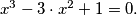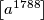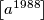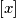### IMO Shortlist 1988 problem 7

Kvaliteta:
Avg: 0,0
Težina:
Avg: 0,0
Let$a$ be the greatest positive root of the equation$x^3 - 3 \cdot x^2 + 1 = 0.$ Show that$\left[a^{1788} \right]$ and$\left[a^{1988} \right]$ are both divisible by 17. Here$[x]$ denotes the integer part of$x.$
Izvor: Međunarodna matematička olimpijada, shortlist 1988